## CodeNewbie Community 🌱Posted on • Originally published at dev.to

# Motivation

Most of the programming languages are open enough to allow programmers to do things multiple ways for a similar outcome. JavaScript is in no way different. With JavaScript, we often find multiple ways of doing things for a similar outcome, and that's confusing at times.

Some of the usages are better than the other alternatives and thus, these are my favorites. I am going to list them here in this article. I am sure, you will find many of these on your list too.

## 1. Forget string concatenation, use template string(literal)

Concatenating strings together using the `+` operator to build a meaningful string is old school. Moreover, concatenating strings with dynamic values(or expressions) could lead to frustrations and bugs.

``````let name = 'Charlse';
let place = 'India';
let isPrime = bit => {
return (bit === 'P' ? 'Prime' : 'Nom-Prime');
}

// string concatenation using + operator
let messageConcat = 'Mr. ' + name + ' is from ' + place + '. He is a' + ' ' + isPrime('P') + ' member.'
``````

Template literals(or Template strings) allow embedding expressions. It has got unique syntax where the string has to be enclosed by the backtick. Template string can contain placeholders for dynamic values. These are marked by the dollar sign and curly braces (\${expression}).

Here is an example demonstrating it,

``````let name = 'Charlse';
let place = 'India';
let isPrime = bit => {
return (bit === 'P' ? 'Prime' : 'Nom-Prime');
}

// using template string
let messageTemplateStr = `Mr. \${name} is from \${place}. He is a \${isPrime('P')} member.`
console.log(messageTemplateStr);
``````

## 2. isInteger

There is a much cleaner way to know if a value is an integer. The `Number` API of JavaScript provides a method called, `isInteger()` to serve this purpose. It is very useful and better to be aware.

``````let mynum = 123;
let mynumStr = "123";

console.log(`\${mynum} is a number?`, Number.isInteger(mynum));
console.log(`\${mynumStr} is a number?`, Number.isInteger(mynumStr));
``````

Output:## 3. Value as Number

Have you ever noticed, `event.target.value` always returns a string type value even when the input box is of type number?

Yes, see the example below. We have a simple text box of type number. It means it accepts only numbers as input. It has an event handler to handle the key-up events.

``````<input type='number' onkeyup="trackChange(event)" />
``````

In the event handler method, we take out the value using `event.target.value`. But it returns a string type value. Now I will have an additional headache to parse it to an integer. What if the input box accepts floating numbers(like, 16.56)? parseFloat() then? Ah, all sorts of confusion and extra work!

``````function trackChange(event) {
let value = event.target.value;
console.log(`is \${value} a number?`, Number.isInteger(value));
}
``````

Use `event.target.valueAsNumber` instead. It returns the value as the number.

``````let valueAsNumber = event.target.valueAsNumber;
console.log(`is \${value} a number?`, Number.isInteger(valueAsNumber));
``````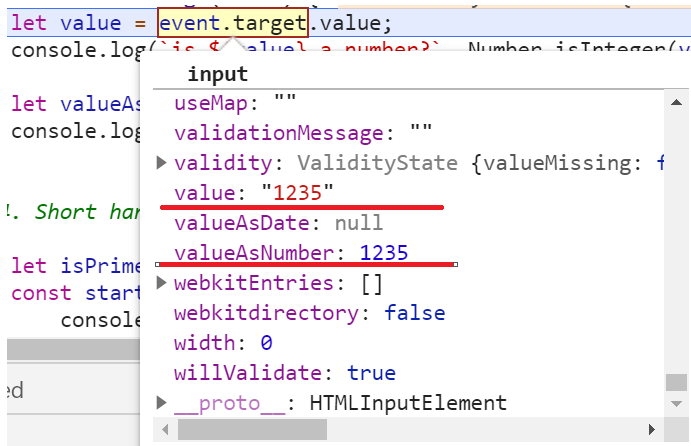## 4. Shorthand with AND

Let's consider a situation where we have a boolean value and a function.

``````let isPrime = true;
const startWatching = () => {
console.log('Started Watching!');
}
``````

This is too much code to check for the boolean condition and invoke the function,

``````if (isPrime) {
startWatching();
}
``````

How about using the short-hand using the AND(&&) operator? Yes, avoid the `if` statement altogether. Cool, right?

``````isPrime && startWatching();
``````

## 5. The default value with || or ??

If you ever like to set a default value for a variable, you can do it using the OR(||) operator easily.

``````let person = {name: 'Jack'};
let age = person.age || 35; // sets the value 35 if age is undefined
console.log(`Age of \${person.name} is \${age}`);
``````

But wait, it has a problem. What if the person's age is 0(a just born baby, maybe). The age will be computed as 35 (`0 || 35 = 35`). This is unexpected behavior.

Enter the `nullish coalescing operator (??)`. It is a logical operator that returns its right-hand side operand when its left-hand side operand is `null` or `undefined`, and otherwise returns its left-hand side operand.

To rewrite the above code with the `??` operator,

``````let person = {name: 'Jack'};
let age = person.age ?? 35; // sets the value 0 if age 0, 35 in case of undefined and null
console.log(`Age of \${person.name} is \${age}`);
``````

## 6. Randoms

Generating a random number or getting a random item from an array is a very useful method to keep handy. I have seen them appearing multiple times in many of my projects.

Get a random item from an array,

``````let planets = ['Mercury ', 'Mars', 'Venus', 'Earth', 'Neptune', 'Uranus', 'Saturn', 'Jupiter'];
let randomPlanet = planets[Math.floor(Math.random() * planets.length)];
console.log('Random Planet', randomPlanet);
``````

Generate a random number from a range by specifying the min and max values,

`````` let getRandom = (min, max) => {
return Math.round(Math.random() * (max - min) + min);
}
console.log('Get random', getRandom(0, 10));
``````

## 7. Function default params

In JavaScript, function arguments(or params) are like local variables to that function. You may or may not pass values for those while invoking the function. If you do not pass a value for a param, it will be `undefined` and may cause some unwanted side effects.

There is a simple way to pass a default value to the function parameters while defining them. Here is an example where we are passing the default value `Hello` to the parameter `message` of the `greetings` function.

``````let greetings = (name, message='Hello,') => {
return `\${message} \${name}`;
}

console.log(greetings('Jack'));
console.log(greetings('Jack', 'Hola!'));
``````

## 8. Required Function Params

Expanding on the default parameter technique, we can mark a parameter as mandatory. First, define a function to throw an error with an error message,

``````let isRequired = () => {
throw new Error('This is a mandatory parameter.');
}
``````

Then assign the function as the default value for the required parameters. Remember, the default values are ignored when a value is passed is as a parameter at the invocation time. But, the default value is considered if the parameter value is `undefined`.

``````let greetings = (name=isRequired(), message='Hello,') => {
return `\${message} \${name}`;
}
console.log(greetings());
``````

In the above code, `name` will be undefined and that will try to set the default value for it which is the `isRequired()` function. It will throw an error as,## 9. Comma Operator

I was surprised when I realized, comma(,) is a separate operator and never gone noticed. I have been using it so much in code but, never realized its true existence.

In JavaScript, the comma(,) operator is used for evaluating each of its operands from left to right and returns the value of the last operand.

``````let count = 1;
let ret = (count++, count);
console.log(ret);
``````

In the above example, the value of the variable `ret` will be, 2. Similar way, the output of the following code will be logging the value 32 into the console.

``````let val = (12, 32);
console.log(val);
``````

Where do we use it? Any guesses? The most common usage of the comma(,) operator is to supply multiple parameters in a for a loop.

``````for (var i = 0, j = 50; i <= 50; i++, j--)
``````

## 10. Merging multiple objects

You may have a need to merge two objects together and create a better informative object to work with. You can use the spread operator `...`(yes, three dots!).

Consider two objects, emp and job respectively,

``````let emp = {
'id': 'E_01',
'name': 'Jack',
'age': 32,
};

let job = {
'title': 'Software Dev',
'location': 'Paris'
};
``````

Merge them using the spread operator as,

`````` // spread operator
let merged = {...emp, ...job};
``````

There is another way to perform this merge. Using `Object.assign()`. You can do it like,

`````` console.log('Object assign', Object.assign({}, emp, job));
``````

Output: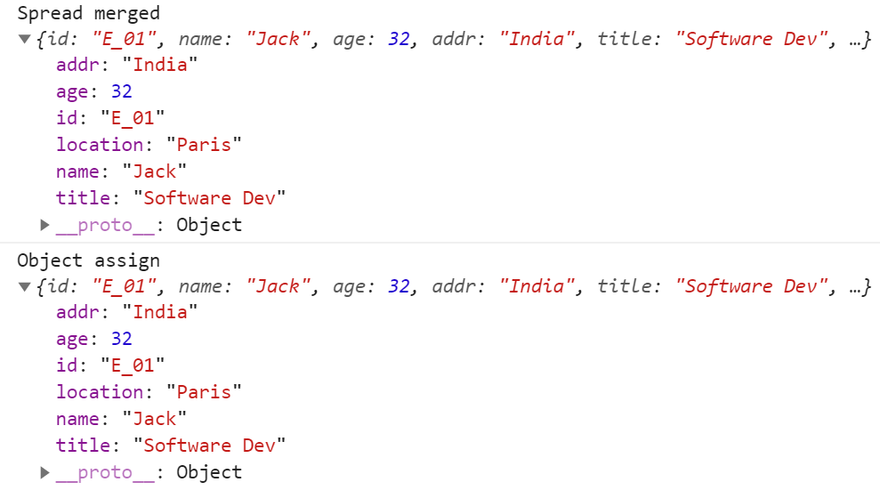Note, both the spread operator and the Object.assign perform a shallow merge. In a shallow merge, the properties of the first object are overwritten with the same property values as the second object.

For deep merge, please use something like, `_merge` of lodash.

## 11. Destructuring

The technique of breaking down the array elements and object properties as variables called, `destructuring`. Let us see it with few examples,

### Array

Here we have an array of emojis,

``````let emojis = ['🔥', '⏲️', '🏆', '🍉'];
``````

To destructure, we would use the syntax as follows,

``````let [fire, clock, , watermelon] = emojis;
``````

This is the same as doing, `let fire = emojis;` but with lots more flexibility.
Have you noticed, I have just ignored the trophy emoji using an empty space in-between? So what will be the output of this?

``````console.log(fire, clock, watermelon);
``````

Output: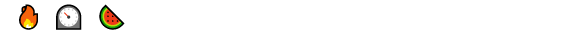Let me also introduce something called the `rest` operator here. If you want to destructure an array such that, you want to assign one or more items to variables and park the rest of it into another array, you can do that using `...rest` as shown below.

``````let [fruit, ...rest] = emojis;
console.log(rest);
``````

Output: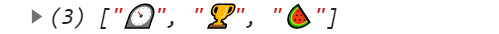### Object

Like arrays, we can also destructure objects.

``````let shape = {
name: 'rect',
sides: 4,
height: 300,
width: 500
};
``````

Destructuring such that, we get a name, sides in a couple of variables and rest are in another object.

``````let {name, sides, ...restObj} = shape;
console.log(name, sides);
console.log(restObj);
``````

Output: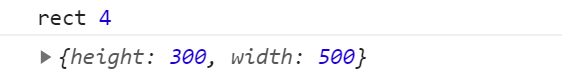## 12. Swap variables

This must be super easy now using the concept of `destructuring` we learned just now.

``````let fire = '🔥';
let fruit = '🍉';

[fruit, fire] = [fire, fruit];
console.log(fire, fruit);
``````

## 13. isArray

Another useful method for determining if the input is an Array or not.

``````let emojis = ['🔥', '⏲️', '🏆', '🍉'];
console.log(Array.isArray(emojis));

let obj = {};
console.log(Array.isArray(obj));
``````

## 14. undefined vs null

`undefined` is where a value is not defined for a variable but, the variable has been declared.

`null` itself is an empty and non-existent value that must be assigned to a variable explicitly.

`undefined` and `null` are not strictly equal,

``````undefined === null // false
``````

## 15. Get Query Params

`window.location` object has a bunch of utility methods and properties. We can get information about the protocol, host, port, domain, etc from the browser URLs using these properties and methods.

One of the properties that I found very useful is,

``````window.location.search
``````

The `search` property returns the query string from the location URL. Here is an example URL: `https://tapasadhiary.com?project=js`. The `location.search` will return, `?project=js`

We can use another useful interface called, `URLSearchParams` along with `location.search` to get the value of the query parameters.

``````let project = new URLSearchParams(location.search).get('project');
``````

Output:
`js`

# This is not the end

This is not the end of the list. There are many many more. I have decided to push those to the git repo as mini examples as and when I encounter them.

# js-tips-tricks

List of JavaScript tips and tricks I am learning everyday!

# Many Thanks to all the `Stargazers` who has supported this project with stars(⭐)